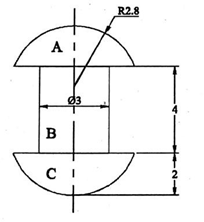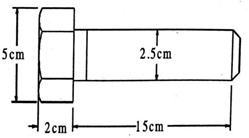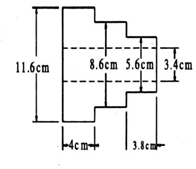### MODEL QUESTION PAPER (CIE) | ESTIMATION & COSTINNG | DIPLOMA 5th and 6th Semester | mechanical engineering

 MODEL QUESTION PAPER (CIE)

 Test/Date and Time Semester/year Course/Course Code Max Marks Ex: I test/6 th weak of sem 10-11 Am VI SEM ESTIMATION & COSTINNG 20 Year: 2016-17 Course code:15ME61T Name of Course coordinator :                                                                                             Units:1,2 Co: 1,2   Note:   Answer all questions Question no Question MARK S CL CO PO 1 Define estimation. List the aims of estimating 05 U 01 2 2 Name the various components of cost. Give brief descriptions of the component. 05 R 02 2 3 A machine was purchased for Rs 30000/-. The cost of erection charges was Rs 10000/- , the estimated life of the machine was 20 years. The scrap value at the end of useful life was Rs 8000/-. Determine the rate of depreciation per year by straight line method. After 10 years the machine underwent major repairs and the repair cost was Rs 6000/- .What will be the new rate of depreciation per year. 10 A 01 1,2

MODEL QUESTION PAPER

VI- Semester Diploma Examination Course Title: ESTIMATION & COSTINNG

Time: 3 Hours]                                                                                            [Max Marks: 100

Note: Answer any SIX from Part A and any SEVEN from Part B

PART-A                                                 6x5=30 marks

1.       Define estimation. Mention the aims of estimating

2.     Explain the Straight line method of calculating depreciation

3.     Name the various components of cost. Give brief descriptions of the component.

4.     Distinguish between fixed and variable overhead

5.     Define cutting speed, feed, depth of cut for lathe operations

6.     Explain the Procedure of estimating cost of machined part for Lathe operations

7.     Explain the various operation time to be considered for estimation of machining time.

8.     Explain the types of sheet metal joints.

9.     Explain the pattern allowances.

PART-B                                       7x10=70 marks

1.     An industrial plant with initial value of Rs. 400000/- has a salvage value of Rs.50000/- at the end of 25 years, but sold for 260000/- at end of 10years. What is the profit or loss to the owner, if sinking fund method of depreciation is adopted? Take interest rate @ 8%.

2.     A catalogue price of a machine is Rs 6000/- and the discount allowed to the distributors is 20% of the catalogue price. At some stage, it was found that the selling expenses were half the factory cost and material cost, labour cost and the factory overheads were in the ratio of 1:4:2 if the material cost was Rs 400/-. What profit was made by the factory on each machine

3.     Determine the number of rivets of dimensions as shown in the figure. Which can be manufactured from 4 Kg of MS. Assume that there is no wastage of material density of MS is 8 gm/cc. All dimensions are in cm.1.     A tapered component is to be manufactured in a lathe by compound rest method out of a given bar of 60mm dia and 100 mm length. Assume the following data:Details of tapered components Major dia =60mmMinor dia =20mm Length of taper =100 mmCutting speed 75 m/min. hand feed by compound rest =0.05 mm/rev depth of cut should not exceed 4 mm. Determine the time required for machining. All dimensions are in mm.

2.     A 20 X 7.5 cm CI surface is to be faced on milling machine with a cutter having a diameter of 125 cm and 20 teeth. If the cutting speed and feed are 50m/min and 4.5 cm/min respectively, determine the milling time, rpm of the cutter and feed per tooth.

3.     Estimate the time required for making an open tank of size 50x50x50 cm by gas welding. Size of sheet used is 50x50x0.3 cm welding is to be done on both sides. Assume fatigue allowance to be 10% and welding speed outside as 6m/hr and inside 5m/hr.

4.     Two 1m long MS plates of 10mm thickness are to be welded by a lap joint with the help of a 6 mm electrode. Determine the cost of welding

a.      Current used                :250 amp

b.     Voltage                        : 30 V

c.      Welding speed             : 10m/hr

d.     Electrode used              : 0.4 kg/m of welding

e.      Labour charges Rs 1/ hr

f.      Power charges Rs 0.20/KWh Cost of electrode Rs 5/kg

5.     Estimate the size of stock and weight of material required to forge 100 MS bolts as shown in figure. The bar stock diameter is 3 cm.1.  Estimate the cost of 2000 CI pulleys as shown in the figure. Its surfaces are to be machined after casting.    The pattern is supplied by the customer itself. Following data can be used:Cost of metal = Rs.5/kg Moulds prepared by each worker/day=20Melting charges            = 20% of metal costMachining allowance on each side may be taken as 2mm Wages of each moulder =Rs. 16/dayDensity of CI = 7.2 gm/cc Overhead charges = 25% of metal cost.10.    a)Explain the procedure for process costing

b) Compare manual tendering with e-tendering